Shortcuts

# Cramer’s V¶

## Module Interface¶

class torchmetrics.CramersV(num_classes, bias_correction=True, nan_strategy='replace', nan_replace_value=0.0, **kwargs)[source]

Compute Cramer’s V statistic measuring the association between two categorical (nominal) data series.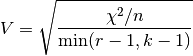where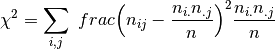where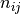denotes the number of times the values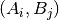are observed with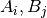represent frequencies of values in preds and target, respectively.

Cramer’s V is a symmetric coefficient, i.e.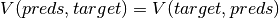.

The output values lies in [0, 1] with 1 meaning the perfect association.

Parameters
Returns

Cramer’s V statistic

Raises
• ValueError – If nan_strategy is not one of ‘replace’ and ‘drop’

• ValueError – If nan_strategy is equal to ‘replace’ and nan_replace_value is not an int or float

Example

>>> from torchmetrics import CramersV
>>> _ = torch.manual_seed(42)
>>> preds = torch.randint(0, 4, (100,))
>>> target = torch.round(preds + torch.randn(100)).clamp(0, 4)
>>> cramers_v = CramersV(num_classes=5)
>>> cramers_v(preds, target)
tensor(0.5284)


Initializes internal Module state, shared by both nn.Module and ScriptModule.

compute()[source]

Computer Cramer’s V statistic.

Return type

Tensor

update(preds, target)[source]

Update state with predictions and targets.

Parameters
• preds (Tensor) – 1D or 2D tensor of categorical (nominal) data

• shape (- _sphinx_paramlinks_torchmetrics.CramersV.update.2D) – (batch_size,)

• shape – (batch_size, num_classes)

target: 1D or 2D tensor of categorical (nominal) data
• 1D shape: (batch_size,)

• 2D shape: (batch_size, num_classes)

Return type

None

## Functional Interface¶

torchmetrics.functional.cramers_v(preds, target, bias_correction=True, nan_strategy='replace', nan_replace_value=0.0)[source]

Compute Cramer’s V statistic measuring the association between two categorical (nominal) data series.wherewheredenotes the number of times the valuesare observed withrepresent frequencies of values in preds and target, respectively.

Cramer’s V is a symmetric coefficient, i.e..

The output values lies in [0, 1] with 1 meaning the perfect association.

Parameters
Return type

Tensor

Returns

Cramer’s V statistic

Example

>>> from torchmetrics.functional import cramers_v
>>> _ = torch.manual_seed(42)
>>> preds = torch.randint(0, 4, (100,))
>>> target = torch.round(preds + torch.randn(100)).clamp(0, 4)
>>> cramers_v(preds, target)
tensor(0.5284)


### cramers_v_matrix¶

torchmetrics.functional.nominal.cramers_v_matrix(matrix, bias_correction=True, nan_strategy='replace', nan_replace_value=0.0)[source]

Compute Cramer’s V statistic between a set of multiple variables.

This can serve as a convenient tool to compute Cramer’s V statistic for analyses of correlation between categorical variables in your dataset.

Parameters
Return type

Tensor

Returns

Cramer’s V statistic for a dataset of categorical variables

Example

>>> from torchmetrics.functional.nominal import cramers_v_matrix
>>> _ = torch.manual_seed(42)
>>> matrix = torch.randint(0, 4, (200, 5))
>>> cramers_v_matrix(matrix)
tensor([[1.0000, 0.0637, 0.0000, 0.0542, 0.1337],
[0.0637, 1.0000, 0.0000, 0.0000, 0.0000],
[0.0000, 0.0000, 1.0000, 0.0000, 0.0649],
[0.0542, 0.0000, 0.0000, 1.0000, 0.1100],
[0.1337, 0.0000, 0.0649, 0.1100, 1.0000]])


© Copyright Copyright (c) 2020-2023, Lightning-AI et al... Revision 825d17f3.

Built with Sphinx using a theme provided by Read the Docs.
Versions
latest
stable
v0.11.3
v0.11.2
v0.11.1
v0.11.0
v0.10.3
v0.10.2
v0.10.1
v0.10.0
v0.9.3
v0.9.2
v0.9.1
v0.9.0
v0.8.2
v0.8.1
v0.8.0
v0.7.3
v0.7.2
v0.7.1
v0.7.0
v0.6.2
v0.6.1
v0.6.0
v0.5.1
v0.5.0
v0.4.1
v0.4.0
v0.3.2
v0.3.1
v0.3.0
v0.2.0
v0.1.0